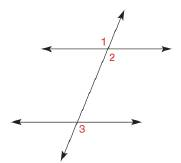Chapter 1.6, Problem 3E### Elementary Geometry for College St...

6th Edition
Daniel C. Alexander + 1 other
ISBN: 9781285195698

#### Solutions

Chapter
Section### Elementary Geometry for College St...

6th Edition
Daniel C. Alexander + 1 other
ISBN: 9781285195698
Textbook Problem
1 views

# In Exercise 3 and 4, supply statements. Given: ∠ 1 ≅ ∠ 2 and ∠ 2 ≅ ∠ 3 Prove: ∠ 1 ≅ ∠ 3 PROOF Statements Reasons 1. ? 1. Given 2. ? 2. Transitive Property of CongruenceTo determine

To prove:

That 13 and give the statements in the table form.

Explanation

Given:

12

23

And,

The given figure is,

Figure (1)

The given table is shown below.

 PROOF Statements Reasons 1. ? 1. Given 2. ? 2. Transitive Property of Congruence

Property used:

The Transitive Property of Congruence states that if two angles are each congruent to a third angle, then they are congruent to each other.

Approach:

The reason for the first statement of the proof is “Given”. So, the given statements are,

12

23

The reason for the second statement of the proof is “Transitive Property of Congruence”.

If two angles are each congruent to a third angle, then they are congruent to each other by the Transitive Property of Congruence.

So, the given statement is,

13

Thus, the table with the statements is shown below

### Still sussing out bartleby?

Check out a sample textbook solution.

See a sample solution

#### The Solution to Your Study Problems

Bartleby provides explanations to thousands of textbook problems written by our experts, many with advanced degrees!

Get Started